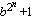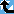# Generalized Fermat Divisors (base=3)

The Prime Pages keeps a list of the 5000 largest known primes, plus a few each of certain selected archivable forms and classes. These forms are defined in this collection's home page.

The numbers Fb,n =(with b an integer greater than one) are called the generalized Fermat numbers. (In the Prime database they are denoted GF(n,b) to avoid the use of subscripts.) It is reasonable to conjecture that for each base b, there are only finitely many such primes.

As in the case of the Fermat numbers, many have interested in the form and distribution of the divisors of these numbers. When b is even, each of their divisors must have the form

k.2m+1
with k odd and m>n. For this reason, when we find a large prime of the form k.2n+1 (with k small), we usually check to see if it divides a Fermat number. For example, Gallot's Win95 program Proth.exe has this test built in for a few choices of b.

The number k.2n+1 (k odd) will divide some generalized Fermat number for roughly 1/k of the bases b.

###Record Primes of this Type

rankprime digitswhowhencomment
13 · 216408818 + 1 4939547 L5171 Oct 2020 Divides GF(16408814, 3), GF(16408817, 5)
29 · 213334487 + 1 4014082 L4965 Mar 2020 Divides GF(13334485, 3)
39 · 212406887 + 1 3734847 L4965 Mar 2020 Divides GF(12406885, 3)
43 · 210829346 + 1 3259959 L3770 Jan 2014 Divides GF(10829343, 3), GF(10829345, 5)
569 · 27452023 + 1 2243285 L4965 Mar 2023 Divides GF(7452020, 3) [GG]
629 · 27374577 + 1 2219971 L5169 Oct 2020 Divides GF(7374576, 3)
73 · 27033641 + 1 2117338 L2233 Feb 2011 Divides GF(7033639, 3)
83 · 25082306 + 1 1529928 L780 Apr 2009 Divides GF(5082303, 3), GF(5082305, 5)
915 · 24800315 + 1 1445040 L1754 Oct 2019 Divides GF(4800313, 3), GF(4800310, 5)
10111 · 24091044 + 1 1231530 L5783 May 2023 Divides GF(4091041, 3)
111065 · 22975442 + 1 895701 L5300 Feb 2021 Divides GF(2975440, 3)
1211 · 22897409 + 1 872209 L2973 Feb 2013 Divides GF(2897408, 3)
1339 · 22705367 + 1 814399 L1576 Apr 2013 Divides GF(2705360, 3)
149 · 22543551 + 1 765687 L1204 Jun 2011 Divides Fermat F(2543548), GF(2543549, 3), GF(2543549, 6), GF(2543549, 12)
153 · 22478785 + 1 746190 g245 Oct 2003 Divides Fermat F(2478782), GF(2478782, 3), GF(2478776, 6), GF(2478782, 12)
163 · 22291610 + 1 689844 L753 Aug 2008 Divides GF(2291607, 3), GF(2291609, 5)
1711 · 22230369 + 1 671410 L2561 Sep 2011 Divides GF(2230368, 3)
183 · 22145353 + 1 645817 g245 Feb 2003 Divides Fermat F(2145351), GF(2145351, 3), GF(2145352, 5), GF(2145348, 6), GF(2145352, 10), GF(2145351, 12)
19753 · 22143388 + 1 645227 L2583 Apr 2014 Divides GF(2143383, 3)
2017 · 21990299 + 1 599141 g267 Mar 2006 Divides GF(1990298, 3)

###References

BR98
A. Björn and H. Riesel, "Factors of generalized Fermat numbers," Math. Comp., 67 (1998) 441--446.  MR 98e:11008 (Abstract available)
DK95
H. Dubner and W. Keller, "Factors of generalized Fermat numbers," Math. Comp., 64 (1995) 397--405.  MR 95c:11010
RB94
H. Riesel and A. Börn, Generalized Fermat numbers.  In "Mathematics of Computation 1943-1993: A Half-Century of Computational Mathematics," W. Gautschi editor, Proc. Symp. Appl. Math. Vol, 48, Amer. Math. Soc., Providence, RI, 1994.  pp. 583-587, MR 95j:11006
Riesel69
H. Riesel, "Some factors of the numbers Gn = 62n + 1 and Hn = 102n + 1," Math. Comp., 23:106 (1969) 413--415.  MR 39:6813